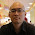### Parallel Matrix Multiply on Hadoop Map/Reduce: 2D blocking

In matrix multiplication, calculating one element of C requires reading an entire row of A and an entire column of B. Calculating an entire column of C (m elements) requires reading all rows (m rows) of A once and re-reading one column of B m times. Thus, Calculating n columns of C requires reading all rows of A n times and all columns of B m times.

In blocking, matrix multiplication of the original arrays is transformed into matrix multiplication of blocks. For example,

C_block(1,1)=A_block(1,1)*B_block(1,1) + A_block(1,2)*B_block(2,1)

This is a well-known Cache Blocking technique for efficient memory accesses. Then, We can think about a Memory Blocking technique for efficient disk accesses and parallelism (or distributed?) on the Hadoop MapReduce as describe below.

Each mapper processes a matrix multiplication of blocks and reducer processes sum up all sub-matrices.

`map(BlockID, TwoDArrayWritable)   -> <C_block(1,1), A_block(1,1)*B_block(1,1)>, ...reduce(BlockID’, <TwoDArrayWritable>*)    -> <C_block(1,1), A_block(1,1)*B_block(1,1) + A_block(1,2)*B_block(2,1)>, ...`

In my own work, It runs greatly. But, I'm not sure it can be auto-optimized.

1.2.